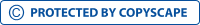Assignment 3 "с Earned Value & ForecastingView larger

# Assignment 3 "с Earned Value & Forecasting

551025

• Ph.D.
• 592

Short excerpt:

Cost Variance (CV) can be calculated as the difference between budgeted cost and actual cost of work performed which is equal to (868,000-1,005,000) i.e. - 137,000. A negative cost variance here implies that the project is over-budget by the end of period 8. The Cost Performance Index (CPI) is given by the ratio of budgeted cost of work performed to the actual cost of work performed i.e. (868,000/1,005,000) which is equal to .86. As the Cost Performance Index is less than 1, it again implies that the project is over-budget.By buying this product you can collect up to 23 loyalty points. Your cart will total 23 loyalty points that can be converted into a voucher of \$0.23.

\$2.37